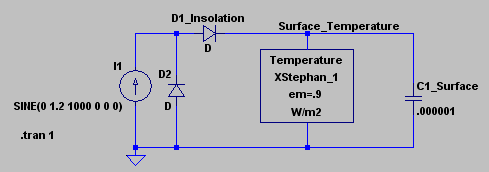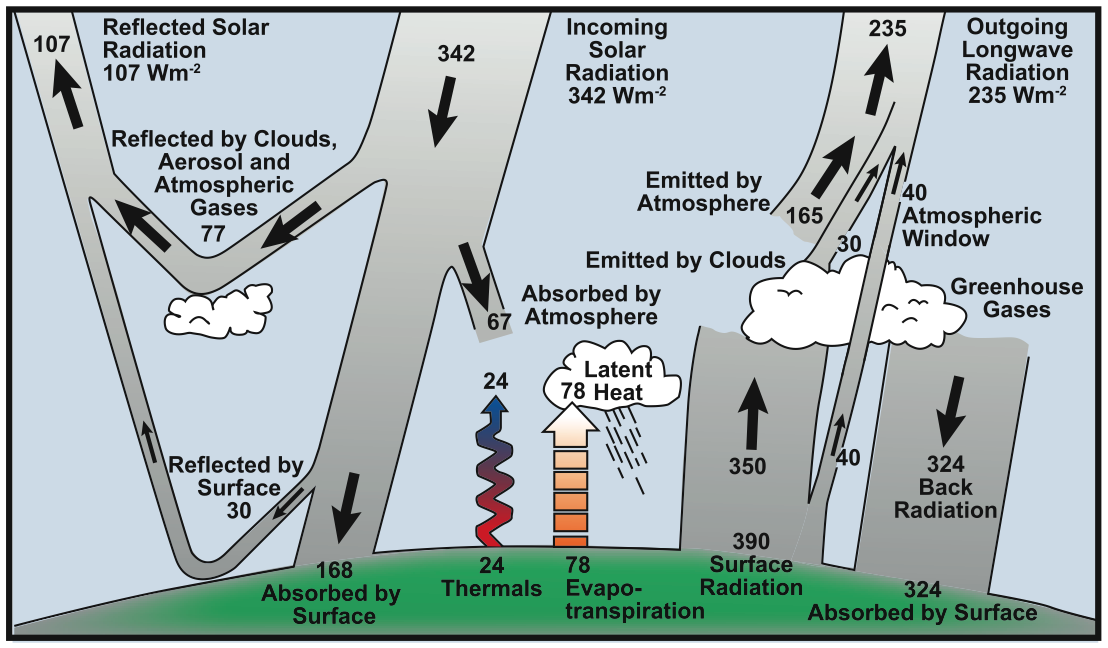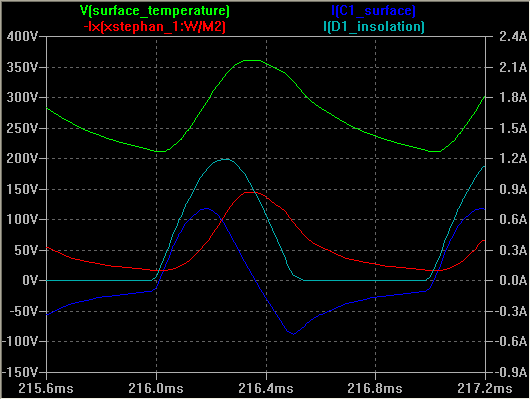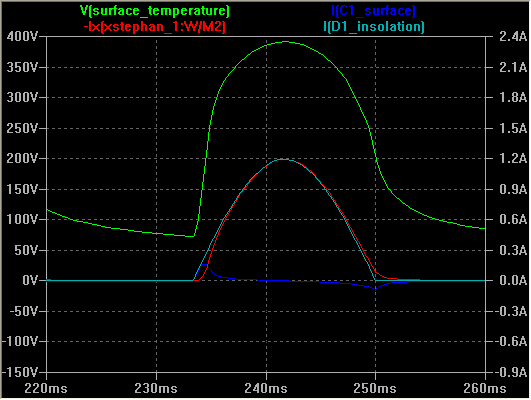### Radiation Balance - LTspice First Look

These pages are related to atmospheric simulation using LTspice. This is a first look at using the Blackbody model and varying the rotation speed.

The solar model (energy source) is simply the positive half of a sine wave with a 50% duty cycle.

The Earth/Moon is modeled with a capacitor and a blackbody radiator.

This model shows the effect of rotation speed on the energy balance of an airless rock in space.

The Model | Greenhouse effect basics | Simulations | Technical details

### The Model

When developing the blackbody radiator component, I needed a way to test it. So I developed a Sun model (a simple half wave rectified sine wave) and added a capacitor to simulate the surface. To get a rapid simulation, I selected 1kHz for the sine wave and an equally random value for the capacitor.The Sun model contains 2 diodes

• D1 simulates day and allows the capacitor to charge
• D2 is required for the simulation to run
Basically, without D2 the simulation takes hours to run, with it, only seconds. I presume that that is because spice is trying to push a very large current backward thru D1. When I tested this with a voltage source, D2 was not required, but the plots were much harder to interpret. A current source more closely models the constant energy coming from the Sun, multiplied by a sine wave to simulate the rotation of the Earth (Moon, etc.). A more advanced model will also account for changes in latitude and seasons, but for a first look a 50% duty cycle is fine.

For the first attempts, the capacitor value was too large - 0.001F, once it obtained full charge there was no appreciable ripple. While this is great for a power supply design, it is a very poor model of the Earth. I simply lowered the value until I got significant ripple, but not enough for it to completely discharge.

A long term goal is to play with the capacitor value to try and match the actual Moon temperatures (which would also verify the model) and then to use that value for the Earth. The difference between the simulated Earth with that value and the actual Earth is, by definition, the greenhouse effect.

### Greenhouse effect basics

To diverge a bit, the primary greenhouse effect is caused by convection. This is almost completely ignored by the Global Warming activists, but it is what keeps the surface temperature from reaching very high values. It limits the maximum surface temperature to about 160°F max. A bit slower to act is evaporation of water - this tends to limit the maximum surface temperature to around 90°F. When there isn't enough water, you tend to get values between these.

Without and atmosphere, the expected maximum temperature on an airless blackbody the same distance from the Sun and the Earth is about 394K (249°F). Albedo and convection reduce that to about 344K (160°F).

The peak temperature is also affected by the height of the Sun in the sky at noon and by the number of hours in the day. These both vary by latitude and season (which is why a future solar model will include them).

As this simple model shows, without an atmosphere the daily minimum temperature due to radiation alone would be well below freezing. This is where the Greenhouse gases return heat from the atmosphere to the surface limiting the minimum nighttime temperature (which the simulation shows actually occurs just after dawn). A simple grey body model (which ignores the atmosphere's absorption spectrum) will help to show how that works.

This other major player in the minimum morning temperature is condensation - dew, fog, and frost. When present, these set the bottom clear sky limit.

Obviously, there is a lot more to it than this - clouds, rain, wind, fronts ..., but these are the basics.

To be clear, most of the charts I've seen show an annual average of the radiation balance. The purpose of these simulations is to investigate the daily and seasonal dynamics. In an annual average, convection and evaporation look pretty trivial, but when presented in these models their significance becomes pretty obvious.source: IPCC AR4 WG1 FAQ-1.1 Fig-1 (similar images) Click link, double click the image, or use mouse wheel to zoom

### Simulations

Earth MoonSimulation of a short day - 1,000 Hz sine wave Simulation of a long day - 30 Hz sine wave
Use the mouse wheel to zoom the images, double click to restore the original size
Key for both images
Image key Color Location on schematic Emulates
V(surface_temperature) green Voltage on the capacitor Temperature of the surface
I(C1_surface) dark blue Current into the capacitor Positive warms the surface, negative cools it
I(D1_insolation) light blue Current thru diode D1 Radiation from the Sun at the surface - zero at night
-Ix(xstephan_1:W/M2) red Minus the current out of the Stephan equation block Radiation from the surface

Be sure to read the voltage (green curve) off the left axis where each volt is one degree kelvin. As an example, 300V corresponds to a temperature of 300K. I know there is no such thing as negative temperature, but I forced the graph to have negative values so that the zeros on the two axes would line up. I think it makes the graphs easier to read.

Currents (the other 3 curves) are read off the right axis where 1.2A corresponds to radiation of 1,200 W/m2.

• Red is the emitted radiation from the surface where emissivity = 0.9
• Light blue is radiation from the sun at right angles (normal) to the surface
• Dark blue is radiation into and out of the surface (capacitor current)

The current into the Stephan equation block and the current out of it are identical except for the sign. In the plots, I selected the output current and multiplied it by minus one so that the W/m2 label would be used in the key - it just seemed the right thing to do.

The only difference in the 2 plots is the frequency of the current source - which simulates the length on the day. The rotation of the Moon is about 28 days, and 1000/30 is about 30 - not very accurate, but for a first look without calibrating the capacitor it is good enough to make some comparisons.

Curves Earth Moon
Temperature (green)
Peak
Minimum

A little over 350K

Almost 400K
Insolation (light blue)
Surface emission (red)
Significant lag Almost identical
Insolation (light blue)
Temperature (green)
There is a significant offset between
noon (peak insolation) and peak temperature
The peaks occur at about the same time
Surface emission (red) Follows the surface temperature Follows the solar energy
Notice that the dark blue curve is the only trace that goes negative. During the day, current (IR power) flows into the capacitor (surface of the Moon) until the peak of the day. After noon on the Moon (or 2 hours after noon for the Earth), the surface cools as it radiates power (negative current).

If you assume a typical atmospheric temperature of 300K (about 80°F), you can see the basic idea of the Greenhouse effect. Assume a straight line at 300v. Where the green curve is above 300v, the area between it and the 300v line represents the excess energy transferred to the atmosphere by convection and evaporation. Where the green curve is below 300v line, the atmosphere is warmer than the surface and Greenhouse gases will return some of the excess heat they received when the green curve was above the line. In this way, the atmosphere acts like a capacitor - storing heat during the day and warming the surface at night.

Astronomers talk about the "Goldilocks zone" - the band around a star where it is not too hot nor too cold for life. Based on this simple model, I think it is obvious that the rotational rate of a planet is also important. Basically, the average daily temperature is irrelevant. However, surviving both the peak and the minimum is very important. Besides the rate of rotation, living below an atmosphere, within an ocean, or under ground will moderate the extremes.

### Technical details

If you want to repeat these runs, this section provides the information you will need.
• Install LTspice - a free electrical circuit simulation package.
• Get FirstLook_01.zip
• Open Stephan_FirstLook.asc in LTspice
• Run the model by clicking on the running man icon (or select Simulate / Run from the menu)

Normally, when you first run the model, no curves will be displayed - on the schematic

• Click on a line (wire) to plot the voltage
• Click on a component to plot the current thru it
However, I have provided 2 *.plt files that automatically load the traces shown in the plots above. As a result, when the simulation is run, the appropriate traces are automatically added - but the axes are the program defaults, not what I used. To get the axes used in the Earth image,
• First click on the plot window to highlight it (this change the available menu selections)
• Then from the menu, select
 ```Plot Settings / Reload Plot Settings ```
When simulating the Moon
• Change the sine wave frequency to 30 Hz
• Run the simulation
• Click on the plot window to highlight it
• Then from the menu, select
 ```Plot Settings / Open Plot Settings File ```
• Select Stephan_FirstLook_moon.plt

There are several values you can edit by right clicking the appropriate symbol on the schematic.

• The frequency and amplitude of the current source (I1 - circle with an arrow pointing up - simulates the Sun)
• The emissivity of the surface - em=0.9 in this model
• The value of the capacitor (C1_surface - simulates heating and cooling the surface - smaller is faster)
• The length of time the simulation covers - .tran 1 simulates 1 second
Since the simulation covers a full second, you will have to zoom the graph to see the details. As an alternative, try 0.01 seconds for an Earth simulation (1,000 Hz) and 0.1 seconds for the Moon (30 Hz).

Useful details from Stephan_FirstLook.net (generated after the first run).

 ```Earth XXStephan_1 Surface_Temperature 0 stephan_bb params: em=.9 C1_Surface Surface_Temperature 0 .000001 I1 0 N001 SINE(0 1.2 1000 0 0 0) Moon XXStephan_1 Surface_Temperature 0 stephan_bb params: em=.9 C1_Surface Surface_Temperature 0 .000001 I1 0 N001 SINE(0 1.2 30 0 0 0) Both .subckt stephan_bb Temperature W/m2 B1 Temperature W/m2 I=(em * 5.67e-8 * V(temperature)**4)/1000 .param em=1 .ends stephan_bb ```
These are the vertical scales for the plots above - automatically set via the plt files.
 ```Left axis V 400 to -150 step 50 Right axis I 2.4 to - 0.9 step 0.3 ```

Author: Robert Clemenzi